An infinite ladder network is arranged with resistances R and 2 R as shown. The effective resistance between terminals A and B is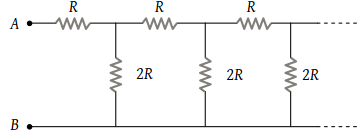(1) ∞

(2) R

(3) 2 R

(4) 3 R

Concept Questions :-

Combination of resistors
High Yielding Test Series + Question Bank - NEET 2020

Difficulty Level:

A battery of emf 10 V and internal resistance 3 Ω is connected to a resistor as shown in the figure. If the current in the circuit is 0.5 A. then the resistance of the resistor will be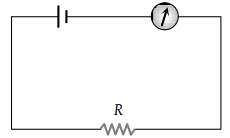(1) 19 Ω

(2) 17 Ω

(3) 10 Ω

(4) 12 Ω

Concept Questions :-

Kirchoff's voltage law
High Yielding Test Series + Question Bank - NEET 2020

Difficulty Level:

The potential drop across the 3Ω resistor is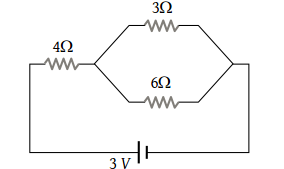(1) 1 V

(2) 1.5 V

(3) 2 V

(4) 3 V

Concept Questions :-

Combination of resistors
High Yielding Test Series + Question Bank - NEET 2020

Difficulty Level:

In the given figure, potential difference between A and B is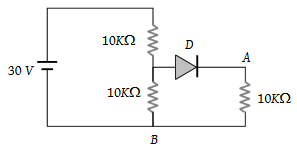(1) 0

(2) 5 volt

(3) 10 volt

(4) 15 volt

Concept Questions :-

Kirchoff's voltage law
High Yielding Test Series + Question Bank - NEET 2020

Difficulty Level:

If each resistance in the figure is of 9 Ω then reading of ammeter is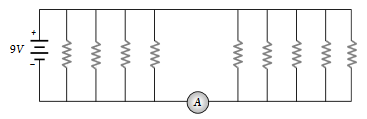(1) 5 A

(2) 8 A

(3) 2 A

(4) 9 A

Concept Questions :-

Combination of resistors
High Yielding Test Series + Question Bank - NEET 2020

Difficulty Level:

Two wires of equal diameters, of resistivities ρ1 and ρ2 and lengths l1 and l2, respectively, are joined in series. The equivalent resistivity of the combination is :

(1) $\frac{{\rho }_{1}{l}_{1}+{\rho }_{2}{l}_{2}}{{l}_{1}+{l}_{2}}$

(2) $\frac{{\rho }_{1}{l}_{2}+{\rho }_{2}{l}_{1}}{{l}_{1}-{l}_{2}}$

(3) $\frac{{\rho }_{1}{l}_{2}+{\rho }_{2}{l}_{1}}{{l}_{1}+{l}_{2}}$

(4) $\frac{{\rho }_{1}{l}_{1}-{\rho }_{2}{l}_{2}}{{l}_{1}-{l}_{2}}$

Concept Questions :-

Derivation of Ohm's law
High Yielding Test Series + Question Bank - NEET 2020

Difficulty Level:

Four resistances of 100 Ω each are connected in the form of square. Then, the effective resistance along the diagonal points is :

(1) 200 Ω

(2) 400 Ω

(3) 100 Ω

(4) 150 Ω

Concept Questions :-

Combination of resistors
High Yielding Test Series + Question Bank - NEET 2020

Difficulty Level:

Equivalent resistance between the points A and B is (in Ω)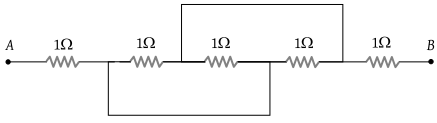(1) $\frac{1}{5}$

(2) $1\frac{1}{4}$

(3) $2\frac{1}{3}$

(4) $3\frac{1}{2}$

Concept Questions :-

Combination of resistors
High Yielding Test Series + Question Bank - NEET 2020

Difficulty Level:

In the circuit shown here, what is the value of the unknown resistor R so that the total resistance of the circuit between points P and Q is also equal to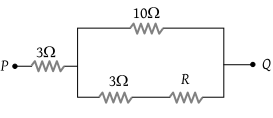(1) 3 ohms

(2) $\sqrt{39}\text{\hspace{0.17em}}ohms$

(3) $\sqrt{69}\text{\hspace{0.17em}}ohms$

(4) 10 ohms

Concept Questions :-

Combination of resistors
High Yielding Test Series + Question Bank - NEET 2020

Difficulty Level:

The resistors of resistances 2 Ω, 4 Ω and 8 Ω are connected in parallel, then the equivalent resistance of the combination will be :

(1) $\frac{8}{7}\Omega$

(2) $\frac{7}{8}\Omega$

(3) $\frac{7}{4}\Omega$

(4) $\frac{4}{9}\Omega$

Concept Questions :-

Combination of resistors